Expressions and Predicates

This chapter discusses general value specifications, known as expressions; and conditional statements, known as predicates.

Expressions

Expressions are used in a variety of contexts within SQL statements, particularly in search condition predicates and the SET clause in UPDATE statements respectively.

An expression always evaluates to a single value.

Syntax

The syntax of an expression is: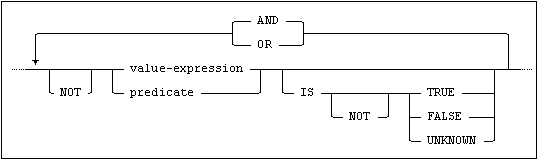where a value-expression is as follows: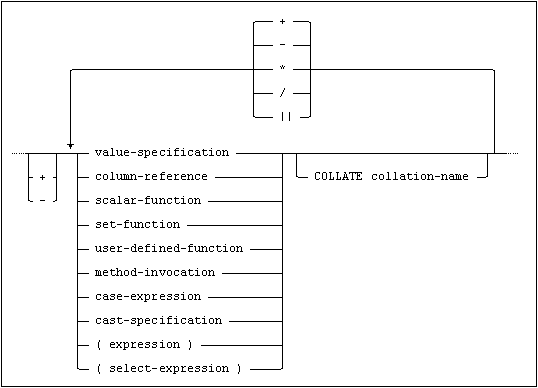Note:A user-defined-function is created by using the CREATE FUNCTION statement.

Note:In this position, the COLLATE clause’s purpose is to specify the result’s collation. E.g. MIN(col_swe) collate english_1 will evaluate MIN according to col_swe’s collation, then the result will have an english_1 collation attribute.

Unary Operators

A unary operator operates on only one operand.

The prefix operator + (unary plus) does not change its operand.

The prefix operator - (unary minus) reverses the sign of its operand.

Binary Operators

A binary operator operates on two operands.

The binary operators specify addition (+), subtraction (-), multiplication (*) and division (/) for numerical operands, and concatenation (||) for string operands.

Operands

When a column name is used as an operand, it represents the single value contained in the column for the row currently addressed when the expression is evaluated.

The column name may be qualified by the name of the table or view, see Identifiers.

Evaluating Arithmetical Expressions

Expressions within parentheses are evaluated first. When the order of evaluation is not specified by parentheses, the customary arithmetical rules apply, i.e. multiplication and division are performed before addition and subtraction and operators with the same precedence are applied from left to right.

If any operand in an expression is null, the whole expression evaluates to null. No other expressions evaluate to null. Division by zero results in a run-time error.

Arithmetical expressions with mixed numerical and character data are illegal.

Note:Where host variables are used in expressions, type conversion may result in apparently incompatible data types being accepted, see Data Types in SQL Statements.

The type and precision of the result of an arithmetical expression is determined in accordance with the rules described below. If there are more than two operands in an expression, the type and precision of the result is derived in accordance with the sequence in which the component binary operations are performed.

Result Data Types

In descriptive terms, the rules are as follows:

If any of the operands is a DOUBLE PRECISION, the result is DOUBLE PRECISION.

For all arithmetic expressions, the result is always a DOUBLE PRECISION, no matter what other data types are involved.

Otherwise, if any of the operands is a REAL, the result is REAL.

For all arithmetic expressions, the result is always a REAL, except for if the other operand is DOUBLE PRECISION, then the result is DOUBLE PRECISION.

Otherwise, if any of the operands is FLOAT(p), the result is FLOAT(p).

For all arithmetic expressions, the precision of the result is the highest operand precision. However, the precision of a FLOAT(p) result is never less than 16.

Otherwise, if all the operands are integer, the result is integer.

The integer data types SMALLINT, INTEGER and BIGINT are used whenever possible (when the result fits).

For addition, subtraction and multiplication with both operands of type SMALLINT, INTEGER or BIGINT, the result data type is the data type of the highest operand, except for operations on two SMALLINT’s, where the result is an INTEGER. For division of SMALLINT, INTEGER or BIGINT, the result data type is the data type of the dividend.

For addition, subtraction and multiplication with at least one operand that is INTEGER(p), where the precision is less than 19, the result data type is an INTEGER or BIGINT, the highest operand decides.

For addition, subtraction and multiplication with at least one operand that is INTEGER(p) where the precision is greater than 18, the result data type is an INTEGER(p). For addition and subtraction, the precision of the result is the precision of the highest operand + 1. For multiplication, the precision of the result is the sum of the precision of the operands. However, the precision may never exceed 45 for the result of any operation.

For division, the result data type is mainly the data type of the dividend. If the dividend is an INTEGER(p < 19), the result data type is a SMALLINT when p < 5, INTEGER when p < 10 or BIGINT when p < 19. If the dividend is an INTEGER(p > 18), the result data type is an INTEGER(p), where the precision is the same as of the dividend.

Otherwise, if all the operands are decimal, or decimal and integer operands are mixed, the result is decimal.

For expressions mixing decimal and integer operands, the integer operand is treated like a decimal with scale set to 0. INTEGER(p) is treated as DECIMAL(p,0), SMALLINT is treated as DECIMAL(5,0), INTEGER is treated as DECIMAL(10,0) and BIGINT is treated as DECIMAL(19,0).

For addition and subtraction, the number of positions to the left of the decimal point (i.e. the difference between precision and scale) in the result is the greatest number of positions in any operand plus 1. The scale of the result is the greatest scale of any of the operands. The precision may not exceed 45.

For multiplication, the precision of the result is the sum of the precisions of the operands.

The scale of the result is the sum of the scales of the operands. Neither the precision nor the scale may exceed 45. If the value of the result does not fit into the precision and scale, overflow occurs.

For division, the precision of the result is the sum of the precisions of the operands. The precision is however never less than 15 and may not exceed 45.

The scale of the result is calculated as the precision of the result, minus the number of positions to the left of the decimal point in the dividend, minus the scale of the divisor.

Evaluating String Expressions

The result of a string concatenation expression is a string containing the first operand string directly followed by the second.

The following rules apply:

If string literals or fixed-length host variables are concatenated, any trailing blanks in the operands are retained.

If a fixed-length character column value is directly concatenated with another string, any trailing blanks in the column value up to the defined fixed length of the column are retained.

If a variable-length character column value is directly concatenated with another string, any trailing blanks in the column value up to the actual length of the column value are retained.

If two character values are concatenated, the result will be a variable-length character value.

If a character value and a national character value are concatenated, the result will be a variable-length national character value.

If either of the operands in a concatenation expression is null, the result of the expression is null.

When concatenating string expressions, the resulting string’s collation depends on whether and where a collation has been specified:

If no collation(s) have been specified for the column-definition, in a domain or explicitly in the concatenation statement, then the resulting string has the Mimer SQL default collation. See Character Sets.

If one string has a specific collation and the other(s) do not then they are coerced into having the specific collation.

If the strings have specific but differing collations, an error will be raised.

Select Specification

A select specification can be used as an expression. This is commonly known as scalar subqueries. A scalar subquery may not return more than one value. The result of an empty subquery is null.

Examples

SET total = (SELECT COUNT(*) FROM categories)

SELECT c.surname, c.forename,

(SELECT COUNT(*) FROM orders

WHERE customer_id = c.customer_id) AS orders

FROM customers AS c

The last example shows a correlated subquery i.e. a subquery with a reference to a column in a table not present in the subquery itself.

CASE Expression

With a CASE expression, it is possible to specify a conditional value. Depending on the result of one or more conditional expressions, the CASE expression can return different values.

A CASE expression can be in one of the following two forms.

CASE Expression First Form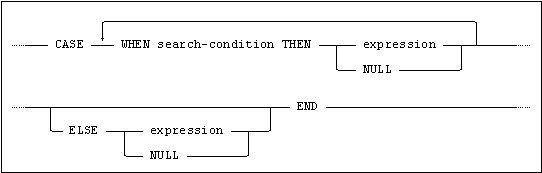Rules

The following rules apply to CASE expressions:

If one or more search-conditions are true, then the result of the CASE expression is the result of the first (left-most) WHEN clause which has a search-condition that is true.

If none of the search-conditions are true, then the result of the CASE expression is the result of the explicit or implicit ELSE clause.

If no ELSE clause is specified then ELSE NULL is implicit.

At least one result in a CASE expression must express a value different from null.

See Result Data Types for a description of how the data type of the result of the CASE expression is determined.

Example

CASE WHEN col1 < 10  THEN 1

WHEN col1 < 100 THEN 2

ELSE 3

END

CASE Expression Second Form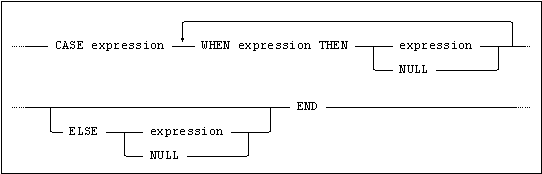Rules

The following rules apply to CASE expressions:

If no ELSE clause is specified then ELSE NULL is implicit.

A case expression using the second form will do an equality comparison between the expression preceding the first when clause and each expression in the when clauses, going from left to right, until one comparison evaluates to true in which case the expression in the THEN part of the when clause is returned. If no comparison evaluates to true, the expression in the else clause is returned.

At least one result in a CASE expression must express a value different from null.

The expression proceeding the first WHEN clause and all expressions in the WHEN clauses must be comparable.

All expressions in the THEN and ELSE clauses must be comparable or be NULL.

See Result Data Types for a description of how the data type of the result of the CASE expression is determined.

Example

CASE col1 WHEN 0 THEN NULL

WHEN -1 THEN -999

ELSE col1

END

Short Forms for CASE

There are two short forms for special CASE expressions: COALESCE and NULLIF.

COALESCE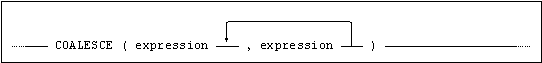where:

COALESCE(x1,x2)

is equivalent to:

CASE WHEN x1 IS NOT NULL THEN x1 ELSE x2

END

and:

COALESCE(x1,x2,...,xn)

is equivalent to:

CASE WHEN x1 IS NOT NULL THEN x1

ELSE COALESCE(x2,...,xn) END

I.e. the COALESCE expression returns the value of the first non-null operand, found by working from left to right, or null if all the operands equal null.

NULLIF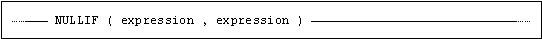where

NULLIF(x1, x2)

is equivalent to

CASE WHEN x1 = x2 THEN NULL ELSE x1 END

I.e. if the operands are equal, the NULLIF expression has the value null, otherwise it has the value of the first operand.

CAST Specification

With the CAST specification it is possible to specify a data type conversion. CAST converts the value of an expression to a specified data type.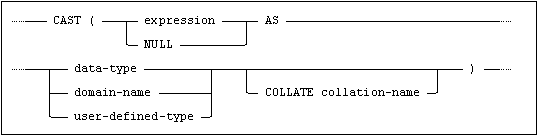Rules

The following rules apply to CAST:

data-type can be any (cast compatible) SQL data type supported by Mimer SQL.

The table below lists cast compatibility.

 Source data type Target data type INTEGER SMALLINT BIGINT INTEGER, BIGINT, SMALLINTINTEGER(p)DECIMAL/NUMERICREAL, DOUBLE PRECISION/FLOATFLOAT(p)CHARACTER, VARCHARNCHAR, NVARCHARBINARY, VARBINARYINTERVAL YEAR-MONTHINTERVAL DAY-TIME INTEGER(p) DECIMAL/NUMERIC INTEGER, BIGINT, SMALLINTINTEGER(p)DECIMAL/NUMERICREAL, DOUBLE PRECISION/FLOATFLOAT(p)CHARACTER, VARCHARNCHAR, NVARCHARINTERVAL YEAR-MONTHINTERVAL DAY-TIME REAL DOUBLE PRECISION/FLOAT FLOAT(p) INTEGER, BIGINT, SMALLINTINTEGER(p)DECIMAL/NUMERICREAL, DOUBLE PRECISION/FLOATFLOAT(p)CHARACTER, VARCHARNCHAR, NVARCHAR CHARACTER VARCHAR INTEGER, BIGINT, SMALLINTINTEGER(p)DECIMAL/NUMERICREAL, DOUBLE PRECISION/FLOATFLOAT(p)CHARACTER, VARCHARNCHAR, NVARCHARBOOLEANBINARY, VARBINARYDATETIMETIMESTAMPINTERVAL YEAR-MONTHINTERVAL DAY-TIME CLOB CLOB NCHAR NVARCHAR INTEGER, BIGINT, SMALLINTINTEGER(p)DECIMAL/NUMERICREAL, DOUBLE PRECISION/FLOATFLOAT(p)CHARACTER, VARCHARNCHAR, NVARCHARBOOLEANDATETIMETIMESTAMPINTERVAL YEAR-MONTHINTERVAL DAY-TIME NCLOB NCLOB DATE DATETIMESTAMPCHARACTER, VARCHARNCHAR, NVARCHAR TIME TIMETIMESTAMPCHARACTER, VARCHARNCHAR, NVARCHAR TIMESTAMP DATETIMETIMESTAMPCHARACTER, VARCHARNCHAR, NVARCHAR INTERVAL YEAR-MONTH INTERVAL YEAR-MONTHINTEGER, BIGINT, SMALLINTINTEGER(p)DECIMAL/NUMERICCHARACTER, VARCHARNCHAR, NVARCHAR INTERVAL DAY-TIME INTERVAL DAY-TIMEINTEGER, BIGINT, SMALLINTINTEGER(p)DECIMAL/NUMERICCHARACTER, VARCHARNCHAR, NVARCHAR BOOLEAN BOOLEANCHARACTER, VARCHARNCHAR, NVARCHAR BINARY VARBINARY BINARY, VARBINARYINTEGER, BIGINT, SMALLINT BLOB BLOB

When converting a value to fixed-length character, the value of the source expression is padded with trailing spaces, if the length of the converted value is shorter than the length of the target data type.

When converting a value to variable-length character, no trailing spaces are padded.

When converting a value to fixed-length binary, the value of the source expression is padded with trailing 0’s, if the length of the converted value is shorter than the length of the target data type.

When converting a value to variable-length binary, no trailing 0’s are padded.

A character value can be converted to a national character value.

A national character value can be converted to a character value. If the national character value contains non-Latin1 characters, an error is raised.

Character values can be converted to a numeric data type if the character string consists of a valid literal representation of the target data type.

Character values can be converted to a DATETIME or INTERVAL data type if the character string consists of a valid literal representation of the target data type.

When a DATE value is converted to a TIMESTAMP, the HOUR, MINUTE and SECOND fields of the target are set to zero. The other fields are set to the corresponding values in the source expression.

When a TIME value is converted to a TIMESTAMP, the respective values for the YEAR, MONTH and DAY fields of the target are obtained by evaluating CURRENT_DATE. The other fields are set to the corresponding values in the source expression.

When a TIMESTAMP value is converted to a DATE or TIME, the fields of the target are set to the corresponding values in the source expression. Any values in the source expression for which there are no corresponding fields in the target are ignored.

When converting from a INTERVAL to an exact numeric value, it must be possible to represent the INTERVAL value as an exact numeric value without the loss of leading significant digits.

When converting from an exact numeric value to an INTERVAL, it must be possible to represent the exact numeric as an INTERVAL value without the loss of leading significant digits.

If CAST is applied on NULL, or if expression results in null, then CAST returns null.

Character values can be converted to a BOOLEAN data type provided expression contains the string TRUE or FALSE regardless of case.

When converting a boolean value to character, the boolean value TRUE is converted to the string TRUE and the boolean value FALSE is converted to the string FALSE.

Example

SELECT CAST(floatcol AS DECIMAL(15,3)),

CAST(charcol AS VARCHAR(10)),

CAST(intcol AS CHAR(15)),

CAST(decimcol AS DOUBLE PRECISION)

FROM types_tab;

User-Defined Function

The syntax to invoke a user-defined function is: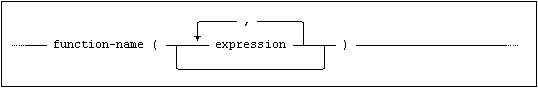The function name may be qualified by a schema name in the normal manner.

Method Invocation

The syntax for a method-invocation is: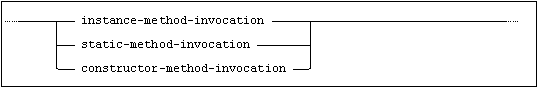and static-method-invocation is: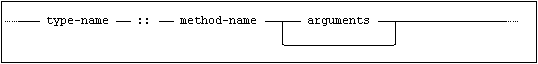and constructor-method-invocation is: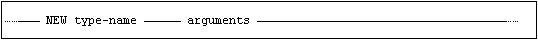and arguments are: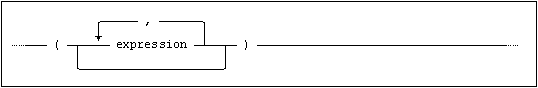The type name may be qualified by a schema name in the normal manner. A method name however can not be qualified by a schema name in the context of a method invocation.

An instance method invocation requires that there is an instance of the user-defined type on which the method is defined. An instance is created by using the initializer function if it is distinct.

Note that if a method invocation returns a user-defined type, it is possible to use this result as an instance for further invocations.

Standard Compliance

This section summarizes standard compliance for expressions.

 Standard Compliance Comments SQL-2016 Core Fully compliant. SQL-2016 Features outside core Feature F052, “Intervals and Datetime arithmetic”. Feature F251, “Domain support” use of domain as target specification in cast expressions. Feature F690, “Collation support” support for collate clause. Feature P002, “Computational completeness”. Feature T031, “Boolean data type”.

Predicates

A predicate is a single conditional expression which evaluates to either true, false or unknown. Predicates are used in constructing search conditions, see Search Conditions.

Predicate Syntax

The general predicate syntax is shown below: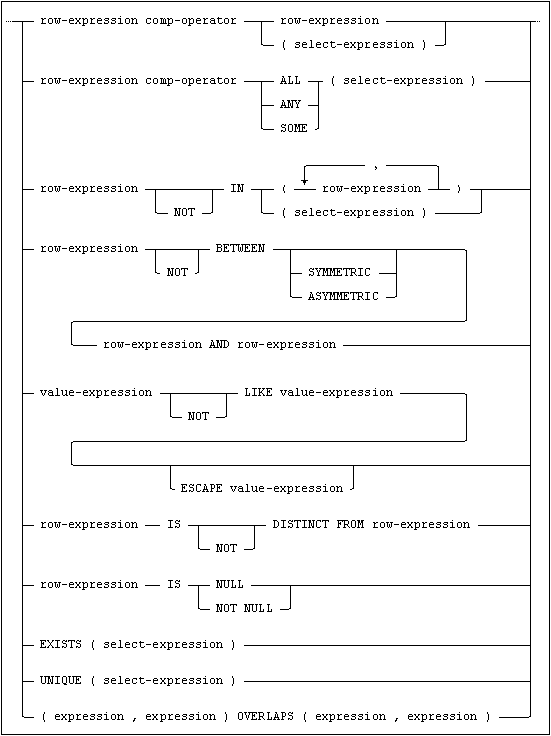where row-expression is: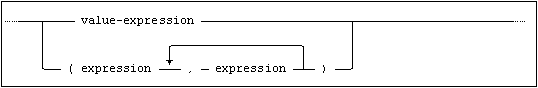Each individual predicate construction is explained in more detail in the following sections.

The Basic Predicate

A basic predicate compares a value with one and only one other value, and has the syntax: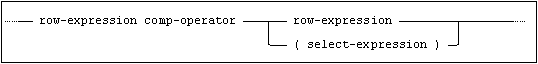The comparison operators, comp-operator, are described in Comparison Operators.

The expressions on either side of the comparison operator must have compatible data types, see Comparisons.

Within the context of a basic predicate, a select-expression must result in either an empty set or a single value.

The result of the predicate is unknown if either of the expressions used evaluates to null, or if the select-expression used results in an empty result set.

A comparison involving row expressions requires that the two row expressions have the same number of elements, and that each element in the first row expression is comparable with the corresponding element in the second row expression.

The comparison will be done from left to right and will continue until all elements have been compared or the predicate is false.

As an example, consider this predicate

(a1,a2,a3) < (b1,b2,b3)

which is equivalent to

a1 < b1 or (a1 <= b1 and a2 < b2) or (a1 <= b1 and a2 <= b2 and a3 < b3)

For instance

(1,date '1956-04-23', false) < (1,date '1956-04-23', true)

would evaluate to TRUE since FALSE is less than TRUE.

Null values are handled analogously with comparisons with single values. Thus

(1,cast(null as int)) < (1,2)

would become null, but

(1,cast(null as int)) < (2,2)

would become true since the second elements are never compared in this case.

Row-expression examples

select * from tabA where (c1, c2) = (select k1, k2 from tabB fetch 1);

select * from tabA where (abs(c1), c2) > (c3, lower(c4));

The Quantified Predicate

A quantified predicate compares an expression with a set of values addressed by a subquery (as opposed to a basic predicate which compares two single-valued expressions).

The form of the quantified expression is: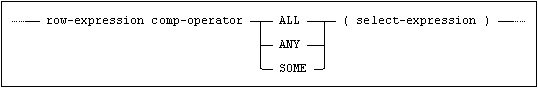The comparison operators, comp-operator, are described in Comparison Operators.

Within the context of a quantified predicate, a select-expression must result in either an empty set or a set of single values.

ALL

The result is true if the select-specification results in an empty set or if the comparison is true for every value returned by the select-expression.

The result is false if the comparison is false for at least one value returned by the select-expression.

The result is unknown if any of the values returned by the select-expression is null and no value is false.

ANY or SOME

The keywords ANY and SOME are equivalent.

The result is true if the comparison is true for at least one value returned by the select-expression.

The result is false if the select results in an empty set or if the comparison is false for every value returned by the select-expression.

The result is unknown if any of the values returned by the select is null and no value is true.

Quantified predicates may always be replaced by alternative formulations using EXISTS, which can often clarify the meaning of the predicates.

The IN Predicate

The IN predicate tests whether a value is contained in a set of discrete values and has the form: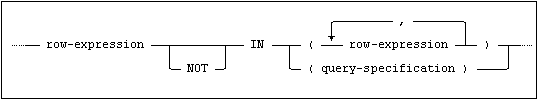If the set of values on the right hand side of the comparison is given as an explicit list, an IN predicate may always be expressed in terms of a series of basic predicates linked by one of the logical operators AND or OR:

IN predicate

Equivalent basic predicates

x IN (a,b,c)

x = a OR x = b OR x = c

x NOT IN (a,b,c)

x <> a AND x <> b AND x <> c

If the set of values is given as a select-expression, an IN predicate is equivalent to a quantified predicate:

IN predicate

Equivalent quantified predicates

x IN (subquery)

x = ANY (subquery)

x NOT IN (subquery)

x <> ALL (subquery)

The result of the IN predicate is unknown if the equivalent predicates give an unknown result.

Note:NOT IN is undefined if the subquery result contains a null value. E.g. SELECT * FROM tab WHERE 3 NOT IN (2, <null>, 4) will return an empty result set.

The BETWEEN Predicate

A BETWEEN predicate tests whether or not a value is within a range of values (including the given limits).

It has the form: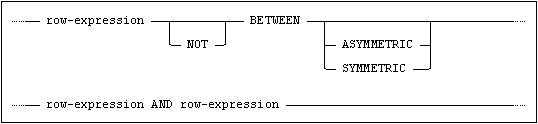The BETWEEN predicate can always be expressed in terms of basic predicates.

If neither SYMMETRIC nor ASYMMETRIC is specified, then ASYMMETRIC is implicit.

Thus:

Between predicate

Equivalent basic predicates

x BETWEEN a AND b

x >= a AND x <= b

x NOT BETWEEN a AND b

x < a OR x > b

x BETWEEN SYMMETRIC a AND b

(x >= a AND x <= b) OR (x >= b AND x <= a)

x NOT BETWEEN SYMMETRIC a AND b

(x > a AND x > b) OR (x < a AND x < b)

Examples

Expression

Result

2 BETWEEN 1 AND 3

TRUE

2 BETWEEN 3 AND 1

FALSE

2 BETWEEN SYMMETRIC 1 AND 3

TRUE

2 BETWEEN SYMMETRIC 3 AND 1

TRUE

(1,2) BETWEEN (1,1) AND (1,3)

TRUE

(1,2) BETWEEN (1,1) AND (1,0)

FALSE

All expressions in the predicate must have compatible data types.

The result of the predicate is unknown if the equivalent basic predicates give an unknown result.

The LIKE Predicate

The LIKE predicate compares the value in a string expression with a character string pattern which may contain wildcard characters (meta-characters).

It has the form: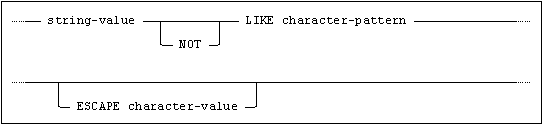The string-value on the left hand side of the LIKE operator must be a string expression.

The character-pattern on the right hand side of the LIKE operator is a string expression.

The escape character-value must be a string expression of length 1. To search for the escape character itself it must appear twice in immediate succession in the like pattern.

Meta-characters/Wildcards

The following meta-characters (wildcards) may be used in the character-pattern:

_   stands for any single character

%   stands for any sequence of zero or more characters.

Note:Wildcard characters are only used as such in LIKE predicates. In any other context, the characters _ and % have their exact values.

Escape Characters

The optional escape character is used to allow matching of the special characters _ and %. When the escape character prefixes _ and %, they are interpreted without any special meaning.

An escape character used in a pattern string may only be followed by another escape character or one of the wildcard characters, unless it is itself escaped (i.e. preceded by an escape character).

Examples

LIKE predicate

Matches

LIKE 'A%'

any string beginning with A

LIKE '_A%'

any string, where the second character is A

LIKE '%A%'

any string containing an A

LIKE '%\%%' ESCAPE '\'

any string containing a %

LIKE '%A\%\\' ESCAPE '\'

any string ending with A%\

LIKE '_ABC'

any 4-character string ending in ABC

A LIKE predicate where the pattern string does not contain any wildcard characters is essentially equivalent to a basic predicate using the = operator.

The comparison strings in the LIKE predicate are not conceptually padded with blanks, in contrast to the basic comparison.

Thus:

'artist  ' = 'artist' is true

'artist  ' LIKE 'artist  ' is true

'artist  ' LIKE 'artist%'   is true

but

'artist  ' LIKE 'artist' is false

Begins With

LIKE predicates, addressing the “begins with” functionality, are very common.

However, when a parameter marker is used for the LIKE pattern, the SQL compiler can not determine the LIKE pattern characteristics, and possible optimizations will not be applied. The built-in function BEGINS will overcome this issue. See BEGINS for information.

Regular Expressions

Compared to LIKE, the regular expression provides a much more flexible way to match strings of text, such as complex patterns of characters.

Use the REGEXP_MATCH function to do regular expression searches. See REGEXP_MATCH for information.

The NULL Predicate

The NULL predicate is used to test if the specified expression is the null value, and has the form: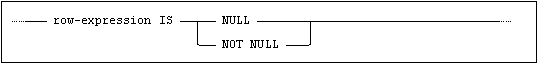The result of the NULL predicate is never unknown.

Evaluation rules for the NULL predicate:

x

x IS NULL

x IS NOT NULL

NOT x IS NULL

NOT x IS NOT NULL

null

True

False

False

True

not null

False

True

True

False

(null, null)

True

False

False

True

(not null, null)

False

False

True

True

(not null, not null)

False

True

True

False

The use of composite expressions in NULL predicates provides a shorthand for testing whether any of the operands is null.

Thus the predicate A=B IS NULL is an alternative to A IS NULL OR B IS NULL.

Note:The actual operator(s) used in expressions in NULL predicates is irrelevant since all operations involving a null value evaluate to the null value.

The NULL predicate is the only way to test for the presence of the null value in a column, since all other predicates where at least one of the operands is null evaluate to unknown.

The EXISTS Predicate

The EXISTS predicate tests whether the set of values addressed by a select-specification is empty or not, and has the form: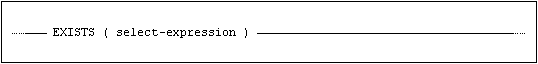The result of the EXISTS predicate is true if the select-expression does not result in an empty set. Otherwise the result of the predicate is false. A set containing only null values is not empty. The result is never unknown.

The EXISTS predicate is the only predicate which does not compare a value with one or more other values. The columns selected in the select-expression of an EXISTS predicate are irrelevant. Most commonly, the SELECT * shorthand is used.

The EXISTS predicate may be negated in the construction of search conditions.

Examples

Consider the four following examples, and note particularly that the last example is true if all guests have undefined names:

Example 1

EXISTS (SELECT * FROM BOOK_GUEST

WHERE GUEST = 'DATE')

requires that at least one guest is called DATE.

Example 2

NOT EXISTS (SELECT * FROM BOOK_GUEST

WHERE GUEST = 'DATE')

requires that no guest may be called DATE.

Example 3

EXISTS (SELECT * FROM BOOK_GUEST

WHERE NOT GUEST = 'DATE')

requires that at least one guest is not called DATE.

Example 4

NOT EXISTS (SELECT * FROM BOOK_GUEST

WHERE NOT GUEST = 'DATE')

requires that no guest may not be called DATE, i.e. every guest must be called DATE (or be null).

The OVERLAPS Predicate

The OVERLAPS predicate tests whether two ‘events’ cover a common point in time or not, and has the form: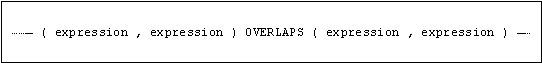Each of the two events specified on either side of the OVERLAPS keyword is a period of time between two specified points on the time-line. The two points can be specified as a pair of datetime values or as one datetime value and an INTERVAL offset.

The first column in each row value expression must be a DATE, TIME or TIMESTAMP and the value in the first column of the first event must be comparable, see Datetime Assignment Rules, to the value in the first column of the second event.

The second column in each row value expression may be either a DATE, TIME or TIMESTAMP that is comparable with the value in the first column or an INTERVAL with a precision that allows it to be added to the value in the first column.

The value in the first column of each row value expression defines one of the points on the time-line for the event.

If the value in the second column of the row value expression is a datetime, it defines the other point on the time-line for the event.

If the value in the second column of the row value expression is an INTERVAL, the other point on the time-line for the event is defined by adding the values in the two column of the row value to expression together.

Either of the two points may be the earlier point in time.

If the value in the first column of the row value expression is the null value, then this is assumed to be the later point in time.

The result of (S1,T1) OVERLAPS (S2,T2) is the result of the following expression:

(S1 > S2 AND NOT (S1 >= T2 AND T1 >= T2))

OR

(S2 > S1 AND NOT (S2 >= T1 AND T2 >= T1))

OR

(S1 = S2 AND (T1 <> T2 OR T1 = T2))

The UNIQUE Predicate

The UNIQUE predicate tests whether all rows returned by a select-specification are unique or not, and has the form: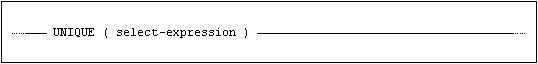The result of the UNIQUE predicate is true if the select-expression does not return any duplicates. Otherwise the result of the predicate is false. The result is never unknown. Null values are not considered equal to any values, including other null values.

The UNIQUE predicate may be negated in the construction of search conditions.

Examples

Return all artists that have only released one item:

SELECT A.*

FROM MIMER_STORE.ARTISTS A

WHERE UNIQUE (SELECT ARTIST_ID

FROM MIMER_STORE_MUSIC.TITLES T

WHERE T.ARTIST_ID = A.ARTIST_ID)

Return all artists that have released items on different formats:

SELECT A.*

FROM MIMER_STORE.ARTISTS A

WHERE NOT UNIQUE (SELECT FORMAT

FROM MIMER_STORE_MUSIC.DETAILS D

WHERE D.ARTIST_ID = A.ARTIST_ID)

The DISTINCT Predicate

The DISTINCT predicate tests whether two values are distinct from each other or not, and has the form: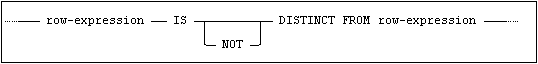If both values are null, the result of the DISTINCT predicate is false. If only one of the values is null, the result of the predicate is true. If none of the values is null, the result of the predicate is true if the values are not the same.

This means that

x IS NOT DISTINCT FROM y

is equivalent to

x = y OR (x IS NULL AND y IS NULL)

And

x IS DISTINCT FROM y

is equivalent to

x <> y OR (x IS NULL AND y IS NOT NULL) OR (x IS NOT NULL AND y IS NULL)

Examples

The following examples are intended to show the difference between distinct from and not equal to when it comes to null values.

Select currencies that have an exchange rate distinct from Sweden’s non-null exchange rate:

SELECT C1.*

FROM MIMER_STORE.CURRENCIES C1

JOIN MIMER_STORE.CURRENCIES C2

ON C1.EXCHANGE_RATE IS DISTINCT FROM C2.EXCHANGE_RATE

WHERE C2.CODE = 'SEK'

The above query will return 161 rows. Countries having a null exchange rate are included.

Select currencies that have an exchange rate not equal to Sweden’s non-null exchange rate:

SELECT c1.*

FROM MIMER_STORE.CURRENCIES C1

JOIN MIMER_STORE.CURRENCIES C2

ON C1.EXCHANGE_RATE <> C2.EXCHANGE_RATE

WHERE C2.CODE = 'SEK'

The above query will return 154 rows. Countries having a null exchange rate are excluded.

Select currencies that have an exchange rate distinct from Saint Helena’s null exchange rate:

SELECT C1.*

FROM MIMER_STORE.CURRENCIES C1

JOIN MIMER_STORE.CURRENCIES C2

ON C1.EXCHANGE_RATE IS DISTINCT FROM C2.EXCHANGE_RATE

WHERE C2.CODE = 'SHP'

The above query will return 151 rows. No countries having a null exchange rate are included.

Select currencies that have an exchange rate not equal to Saint Helena’s null exchange rate:

SELECT COUNT(*)

FROM MIMER_STORE.CURRENCIES C1

JOIN MIMER_STORE.CURRENCIES C2

ON C1.EXCHANGE_RATE <> C2.EXCHANGE_RATE

WHERE C2.CODE = 'SHP'

The above query will return 0 rows.

Standard Compliance

This section summarizes standard compliance concerning predicates.

Standard

Compliance

SQL-2016

Core

Fully compliant.

SQL-2016

Features outside core

Feature F052, “Intervals and datetime arithmetic” overlaps predicate.

Feature F053, “OVERLAPS predicate”.

Feature F281, “LIKE enhancements”. The arguments for a LIKE predicate may be value expressions.

Feature F291, “UNIQUE predicate”.

Feature F561, “Full value expression”.

Feature F641, “Row and table constructors”.

Feature T022, “Advanced support for BINARY and VARBINARY data types”.

Feature T151, “DISTINCT predicate”.

Feature T152, “DISTINCT predicate with negation”.

Feature T461, “Symmetric BETWEEN predicate”.

Feature T501, “Enhanced EXISTS predicate”.